Subsections

# Landau Theory

## Lifshits Term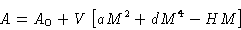Now we divide system into layers, andwith A(x) being the free energy of one layer.

We want to add the dependence on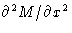. Lowest order: linear dependence on. But this is not symmetric with respect to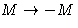. Solution: use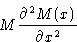Integrating by parts: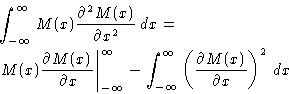(2)
If there is bulk phase at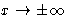, first term is zero. Otherwise we can include it in the boundary conditions. Result: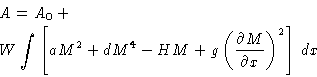where W is the cross-section normal to x

General case: substitute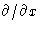by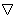: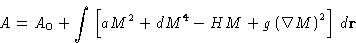(3)
The term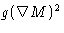is called Lifshits term, and free energy (3)--Landau Hamiltonian (or Landau-Ginsburg Hamiltonian).

## Estimates for Lifshits Term

1.
Sign of g: if g<0, wavy'' structures are favored:Therefore g>0.

2.
Value of g: the dimensionality of g/d is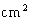(Why?). From previous section it is clear, that this of the order of the square of the molecular size a0: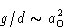Since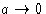at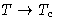, we obtain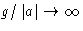. We will see that this is square of the correlation length: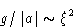Next: Mathematical Digression: Euler-Lagrange Equation Up: Spatial Inhomogeneity. Interfaces Previous: Self-Consistent Field Theory

© 1997 Boris Veytsman and Michael Kotelyanskii
Thu Oct 16 20:58:44 EDT 1997